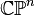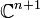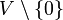# Complex projective space

Jump to: navigation, search

## Definition

### Short definition

Complex projective space is defined as projective space over a field of complex numbers$\mathbb{C}$, where the topological structure is induced from the structure of the field of complex numbers as a topological field.

### Finite-dimensional

Complex projective space of dimension$n$, denoted$\mathbb{C}\mathbb{P}^n$ or$\mathbb{P}^n(\mathbb{C})$, is defined as the quotient space under the group action$\mathbb{C}^{n+1} \setminus \{ 0 \}/\mathbb{C}^*$ where$\mathbb{C}^*$ acts by scalar multiplication. It is equipped with the quotient topology.

As a set, we can think of it as the set of complex lines (which are planes in the real vector space sense) through the origin in$\mathbb{C}^{n+1}$. Using a Hermitian inner product on$\mathbb{C}^{n+1}$, it can also be identified with the set of hyperplanes of codimension 1 (i.e.,$n$-dimensional complex linear subspaces) in$\mathbb{C}^{n+1}$.

### Countable-dimensional

This space, called countable-dimensional complex projective space and denoted$\mathbb{C}\mathbb{P}^\infty$, is defined as the quotient space of the nonzero elements of a countable-dimensional complex vector space (with the standard topology) over$\mathbb{C}$ by the action of$\mathbb{C}^*$ by scalar multiplication.

### Others

We can also consider the real projective space corresponding to any topological complex vector space, possibly infinite-dimensional, which is a real vector space equipped with a compatible topology. If the vector space is$V$, the projective space is defined as follows:we take$V \setminus \{ 0 \}$ with the subspace topology, and then put the quotient topology on its quotient under the action of$\mathbb{C}^*$.

## Particular cases$n$ Complex projective space$\mathbb{C}\mathbb{P}^n$
0 one-point space
1 complex projective line, which turns out to be homeomorphic to the 2-sphere
2 complex projective plane
3 link: Fill this in later
countable ($\infty$) countable-dimensional complex projective space

## Algebraic topology

### Homology

Further information: homology of complex projective space

### Cohomology

Further information: cohomology of complex projective space

### Homotopy

Further information: homotopy of complex projective space